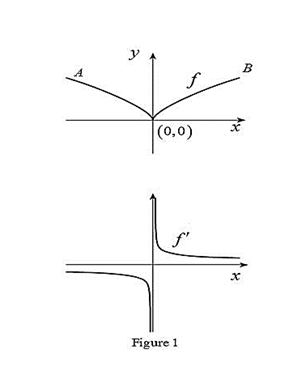# The graph of f ′ below the graph of f.### Single Variable Calculus: Concepts...

4th Edition
James Stewart
Publisher: Cengage Learning
ISBN: 9781337687805### Single Variable Calculus: Concepts...

4th Edition
James Stewart
Publisher: Cengage Learning
ISBN: 9781337687805

#### Solutions

Chapter 2.7, Problem 8E
To determine

## To sketch: The graph of f′ below the graph of f.

Expert Solution

### Explanation of Solution

From the point A to the left, the slope of the graph f is strictly negative which implies that the derivative graph f must have a functional value in negative.

From the given graph, it is observed that the graph has high sharpness at origin. So, there is no unique tangent line at origin. Thus, the given graph is not differentiable at (0,0). Therefore, the graph of f has a discontinuity at x=0.

From the point A to right, the slope of the graph f is strictly positive which implies that the derivative graph must have a functional value in positive.

Graph:

Use the above information and obtain the graph of f(x) as shown below in Figure 1.Thus, f is the required graph.

### Have a homework question?

Subscribe to bartleby learn! Ask subject matter experts 30 homework questions each month. Plus, you’ll have access to millions of step-by-step textbook answers!